# BMAT: Section 2 Mathematics [The Definitive Guide]

## Format Of BMAT Section 2

In Section 2, there are a total of 27 questions to answer in 30 minutes. This leaves you with approximately 1 minute per question.

Section 2 is the most time-pressured section. Therefore it is very important to ensure you know your material well.

We would recommend doing past papers under timed conditions so you get used to the time pressure.

Concerning maths, you will face 6 purely mathematics questions, however, there will be maths integrated into the other questions as well so make sure your mental maths is up to scratch.

This is how many questions are on each topic:

TopicNumber Of Questions
Biology7
Chemistry7
Physics7
Mathematics6
Total27

Section 2 is scored from 1-9 with 9 being the best score you can get.

It’s important to note that barely any candidates each year score 8 or above. A score of 6 or above is good!

## BMAT Section 2: Maths Specification

This is your guide to the Maths topics that may be asked of you. Every topic is outlined below.

If you are wanting specific details on them, this can be found on the official BMAT specification.

Here are the following topics you should be prepared for. Just click the topic below to expand the information.

Alternatively, if you’re comfortable with the specification and just want to see some fully worked questions, just click here.

 M1.1 Use standard units of mass, length, time, money and other measures. Use compound units such as speed, rates of pay, unit pricing, density and pressure, including using decimal quantities where appropriate. M1.2 Change freely between related standard units (e.g. time, length, area, volume/capacity, mass) and compound units (e.g. speed, rates of pay, prices, density, pressure) in numerical and algebraic contexts.

See a fully worked Numbers question and answer here.

 M2.1 Order positive and negative integers, decimals and fractions. Understand and use the symbols: = , ≠ , < , > , ≤ , ≥ . M2.2 Apply the four operations (addition, subtraction, multiplication and division) to integers, decimals, simple fractions (proper and improper) and mixed numbers – any of which could be positive and negative. Understand and use place value. M2.3 Use the concepts and vocabulary of prime numbers, factors (divisors), multiples, common factors, common multiples, highest common factor, lowest common multiple, and prime factorisation (including use of product notation and the unique factorisation theorem). M2.4 Recognise and use relationships between operations, including inverse operations. Use cancellation to simplify calculations and expressions. Understand and use the convention for priority of operations, including brackets, powers, roots and reciprocals. M2.5 Apply systematic listing strategies. (For instance, if there are m ways of doing one task and for each of these tasks there are n ways of doing another task, then the total number of ways the two tasks can be done in order is m× n ways.) M2.6 Use and understand the terms: square, positive and negative square root, cube and cube root. M2.7 Use index laws to simplify numerical expressions, and for multiplication and division of integer, fractional and negative powers. M2.8 Interpret, order and calculate with numbers written in standard index form (standard form); numbers are written in standard form as a × 10n, where 1 ≤ a < 10 and n is an integer. M2.9 Convert between terminating decimals, percentages and fractions. Convert between recurring decimals and their corresponding fractions M2.10 Use fractions, decimals and percentages interchangeably in calculations. Understand equivalent fractions. M2.11 Calculate exactly with fractions, surds and multiples of π. Simplify surd expressions involving squares, e.g. √12 = √4 x 3 = √4√3 = 2√3, and rationalise denominators; for example, candidates could be asked to rationalise expressions such as: 3 / √7, 5 / 3 + 2√5, 7 / 2 – √3, 3 / √5 – √2. M2.12 Calculate with upper and lower bounds, and use in contextual problems. M2.13 Round numbers and measures to an appropriate degree of accuracy, e.g. to a specified number of decimal places or significant figures. Use inequality notation to specify simple error intervals due to truncation or rounding. M2.14 Use approximation to produce estimates of calculations, including expressions involving π or surds.

See a fully worked Ratio & Proportion question and answer here.

 M3.1 Understand and use scale factors, scale diagrams and maps. M3.2 Express a quantity as a fraction of another, where the fraction is less than 1 or greater than 1. M3.3 Understand and use ratio notation. M3.4 Divide a given quantity into two (or more) parts in a given part:part ratio. Express the division of a quantity into two parts as a ratio. M3.5 Apply ratio to real contexts and problems, such as those involving conversion, comparison, scaling, mixing and concentrations. Express a multiplicative relationship between two quantities as a ratio or a fraction. M3.6 Understand and use proportion. Relate ratios to fractions and to linear functions. M3.7 Identify and work with fractions in ratio problems. M3.8 Define percentage as ‘number of parts per hundred’. Interpret percentages and percentage changes as a fraction or a decimal, and interpret these multiplicatively. Express one quantity as a percentage of another. Compare two quantities using percentages. Work with percentages greater than 100%. Solve problems involving percentage change, including percentage increase/decrease, original value problems and simple interest calculations. M3.9 Understand and use direct and inverse proportion, including algebraic representations. Recognise and interpret graphs that illustrate direct and inverse proportion. Set up, use and interpret equations to solve problems involving direct and inverse proportion (including questions involving integer and fractional powers). Understand that x is inversely proportional to y is equivalent to x is proportional to 1 / y. M3.10 Compare lengths, areas and volumes using ratio notation. Understand and make links to similarity (including trigonometric ratios) and scale factors M3.11 Set up, solve and interpret the answers in growth and decay problems, including compound interest, and work with general iterative processes.

See a fully worked Algebra question and answer here.

 M4.1 Understand, use and interpret algebraic notation; for instance: ab in place of a×b ; 3y in place of y+y+y and 3 × y ; a2 in place of a×a ; a3 in place of a×a×a ; a2b in place of a×a×b ; a / b in place of a ÷ b M4.2 Use index laws in algebra for multiplication and division of integer, fractional, and negative powers. M4.3 Substitute numerical values into formulae and expressions, including scientific formulae. Understand and use the concepts and vocabulary: expressions, equations, formulae, identities, inequalities, terms and factors. M4.4 Collect like terms, multiply a single term over a bracket, take out common factors, and expand products of two or more binomials. M4.5 Factorise quadratic expressions of the form x2 + bx +c, including the difference of two squares. Factorise quadratic expressions of the form ax2 + bx +c, including the difference of two squares. M4.6 Simplify expressions involving sums, products and powers, including the laws of indices. Simplify rational expressions by cancelling, or factorising and cancelling. Use the four rules on algebraic rational expressions. M4.7 Rearrange formulae to change the subject. M4.8 Understand the difference between an equation and an identity. Argue mathematically to show that algebraic expressions are equivalent M4.9 Work with coordinates in all four quadrants. M4.10 Identify and interpret gradients and intercepts of linear functions ( y = mx + c ) graphically and algebraically. Identify pairs of parallel lines and identify pairs of perpendicular lines, including the relationships between gradients. Find the equation of the line through two given points, or through one point with a given gradient. M4.11 Identify and interpret roots, intercepts and turning points of quadratic functions graphically. Deduce roots algebraically, and turning points by completing the square. M4.12 Recognise, sketch and interpret graphs of: a. linear functions b. quadratic functions c. simple cubic functions d. the reciprocal function: y = x / 1 with x≠0 e. the exponential function: y = kx for positive values of k f. trigonometric functions (with arguments in degrees): y = sin x, y = cos x, y = tan x for angles of any size M4.13 Interpret graphs (including reciprocal graphs and exponential graphs) and graphs of non-standard functions in real contexts to find approximate solutions to problems, such as simple kinematic problems involving distance, speed and acceleration. M4.14 Calculate or estimate gradients of graphs and areas under graphs (including quadratic and other non-linear graphs), and interpret results in cases such as distance–time graphs, speed–time graphs and graphs in financial contexts. M4.15 Set up and solve, both algebraically and graphically, simple equations including simultaneous equations involving two unknowns; this may include one linear and one quadratic equation. Solve two simultaneous equations in two variables (linear/linear or linear/quadratic) algebraically. Find approximate solutions using a graph. Translate simple situations or procedures into algebraic expressions or formulae; for example, derive an equation (or two simultaneous equations), solve the equation(s) and interpret the solution. M4.16 Solve quadratic equations (including those that require rearrangement) algebraically by factorising, by completing the square, and by using the quadratic formula. Know the quadratic formula: x = – b ± √b2 – 4ac / 2a Find approximate solutions of quadratic equations using a graph. M4.17 Solve linear inequalities in one or two variables. Represent the solution set on a number line, or on a graph, or in words. M4.18 Generate terms of a sequence using term-to-term or position-to-term rules. M4.19 Deduce expressions to calculate the nth term of linear or quadratic sequences.

See a fully worked Geometry question and answer here.

 M5.1 Use conventional terms and notation: points, lines, line segments, vertices, edges, planes, parallel lines, perpendicular lines, right angles, subtended angles, polygons, regular polygons and polygons with reflection and/or rotational symmetries. M5.2 Recall and use the properties of angles at a point, angles on a straight line, perpendicular lines and opposite angles at a vertex. Understand and use the angle properties of parallel lines, intersecting lines, triangles and quadrilaterals. Calculate and use the sum of the interior angles, and the sum of the exterior angles, of polygons. M5.3 Derive and apply the properties and definitions of special types of quadrilaterals, including square, rectangle, parallelogram, trapezium, kite and rhombus. Derive and apply the properties and definitions of various types of triangle and other plane figures using appropriate language. M5.4 Understand and use the basic congruence criteria for triangles (SSS, SAS, ASA, RHS). M5.5 Apply angle facts, triangle congruence, similarity, and properties of quadrilaterals to results about angles and sides. M5.6 Identify, describe and construct congruent and similar shapes, including on coordinate axes, by considering rotation, reflection, translation and enlargement (including fractional and negative scale factors). Describe the changes and invariance achieved by combinations of rotations, reflections and translations. Describe translations as 2-dimensional vectors. M5.7 Know and use the formula for Pythagoras’ theorem: a2 + b2 = c2 Use Pythagoras’ theorem in both 2 and 3 dimensions. M5.8 Identify and use conventional circle terms: centre, radius, chord, diameter, circumference, tangent, arc, sector and segment (including the use of the terms minor and major for arcs, sectors and segments). M5.9 Apply the standard circle theorems concerning angles, radii, tangents and chords, and use them to prove related results: a. angle subtended at the centre is twice the angle subtended at the circumference b. angle in a semicircle is 90° c. angles in the same segment are equal d. angle between a tangent and a chord (alternate segment theorem) e. angle between a radius and a tangent is 90° f. properties of cyclic quadrilaterals M5.10 Solve geometrical problems on 2-dimensional coordinate axes. M5.11 Know the terminology faces, surfaces, edges and vertices when applied to cubes, cuboids, prisms, cylinders, pyramids, cones, spheres and hemispheres. M5.12 Interpret plans and elevations of 3-dimensional shapes. M5.13 Use and interpret maps and scale drawings. Understand and use three-figure bearings. M5.14 Know and apply formulae to calculate: a. the area of triangles, parallelograms, trapezia b. the volume of cuboids and other right prisms. M5.15 Know the formulae: a. circumference of a circle = 2πr = πd b. area of a circle = πr2 c. volume of a right circular cylinder = πr2h Formulae relating to spheres, pyramids and cones will be given if needed. Use formulae to calculate: a. perimeters of 2-dimensional shapes, including circles b. areas of circles and composite shapes c. surface area and volume of spheres, pyramids, cones and composite solids M5.16 Calculate arc lengths, angles and areas of sectors of circles. M5.17 Apply the concepts of congruence and similarity in simple figures, including the relationships between lengths, areas and volumes. M5.18 Know and use the trigonometric ratios: sinθ = opposite / hypotenuse cosθ = adjacent / hypotenuse tanθ = opposite / adjacent Apply these to find angles and lengths in right-angled triangles and, where possible, general triangles in 2- and 3-dimensional figures. Know the exact values of sinθ and cosθ for θ = 0°, 30°, 45°, 60°, 90°. Know the exact values of tanθ for θ = 0°, 30°, 45°, 60°. Candidates are not expected to recall or use the sine or cosine rules. M5.19 Apply addition and subtraction of vectors, multiplication of vectors by a scalar, and diagrammatic and column representations of vectors. Use vectors to construct geometric arguments and proofs.

See a fully worked Statistics question and answer here.

 M6.1 Interpret and construct tables, charts and diagrams, including: a. two-way tables, frequency tables, bar charts, pie charts and pictograms for categorical data b. vertical line charts for ungrouped discrete numerical data c. tables and line graphs for time series data Know the appropriate use of each of these representations. M6.2 Interpret and construct diagrams for grouped discrete data and continuous data: a. histograms with equal and unequal class intervals b. cumulative frequency graphs Know the appropriate use of each of these diagrams. Understand and use the term frequency density. M6.3 Calculate the mean, mode, median and range for ungrouped data. Find the modal class; calculate estimates of the range, mean and median for grouped data, and understand why these are estimates. Describe a population using statistics. Make simple comparisons. Compare data sets using like-for-like summary values. Understand the advantages and disadvantages of summary values. Calculate estimates of mean, median, mode, range, quartiles and interquartile range from graphical representation of grouped data. Use the median and interquartile range to compare distributions. M6.4 Use and interpret scatter graphs of bivariate data. Recognise correlation, and know that it does not indicate causation. Draw estimated lines of best fit. Interpolate and extrapolate apparent trends whilst knowing the dangers of so doing.

See a fully worked Probability question and answer here.

 M6.1 Analyse the frequency of outcomes of probability experiments using tables and frequency trees. M6.2 Apply ideas of randomness, fairness and equally likely events to calculate expected outcomes of multiple future experiments. Understand that if an experiment is repeated, the outcome may be different. M6.3 Relate relative expected frequencies to theoretical probability, using appropriate language and the ‘0 to 1’ probability scale. M6.4 Apply the property that the probabilities of an exhaustive set of outcomes sum to one. Apply the property that the probabilities of an exhaustive set of mutually exclusive events sum to one. M6.5 Enumerate sets and combinations of sets systematically, using tables, grids, Venn diagrams and tree diagrams. Candidates are not expected to know formal set theory notation. M6.6 Construct theoretical possibility spaces for single and combined experiments with equally likely outcomes, and use these to calculate theoretical probabilities. M6.7 Know when to add or multiply two probabilities, and understand conditional probability. Calculate and interpret conditional probabilities through representation using expected frequencies with two-way tables, tree diagrams and Venn diagrams. Understand the use of tree diagrams to represent outcomes of combined events: a. when the probabilities are independent of the previous outcome b. when the probabilities are dependent on the previous outcome.

You are also expected to be familiar with the following SI prefixes:

Prefix NameValue
Nano-10-9
Micro-10-6
Milli-10-3
Centi-10-2
Deci-10-1
Kilo-103
Mega-106
Giga-109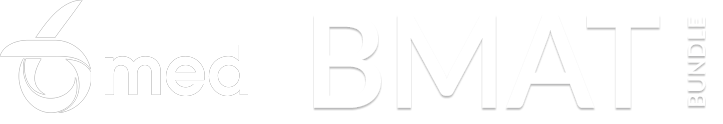## BMAT Maths Fully Worked Questions & Answers For Every Topic

In this section we’ll go through a fully worked solution for every topic in the BMAT Maths specification.

If you want to work through the questions yourself first scroll with care!

### Numbers: Fully Worked Question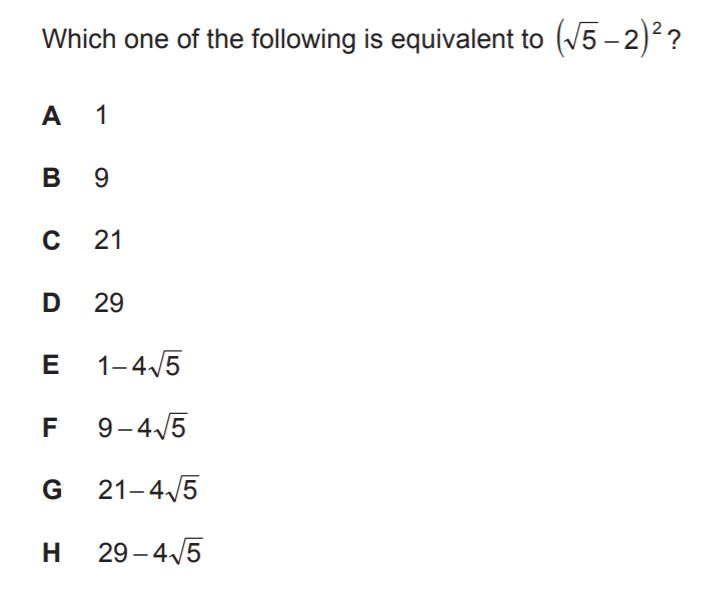### Numbers: Fully Worked Solution

In this question, they want you to expand the bracket and then simplify the expression.

This question requires you knowing that a surd squared gives you the number within the surd. Therefore 2 is 5. It also requires you to know that two minus numbers squared will give you a positive.

Expanding the bracket gives us 5 – 2 – 2 + 4 which gives us the answer F.

### Ratio & Proportion: Fully Worked Question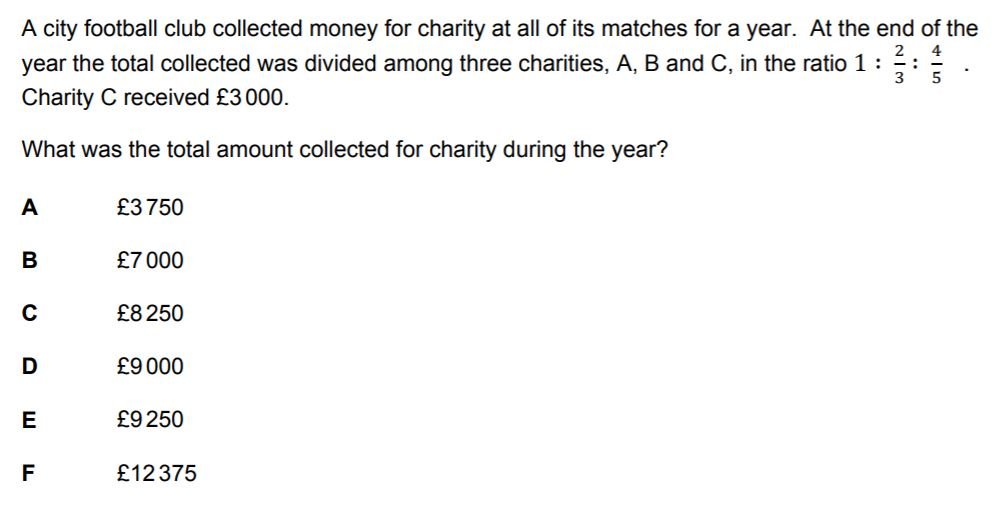### Ratio & Proportion: Fully Worked Solution

This question is about ratios. To tackle this, start by finding a common factor of the fractions. In this case, it is 15. The ratio can be expressed as 15/15 : 10/15 : 12/15.

If  12/15 equals £3000 then 10/15 will equal £2500 and 15/15 is £3750, giving a total of £9250.

This makes the correct answer (E).

### Algebra: Fully Worked Question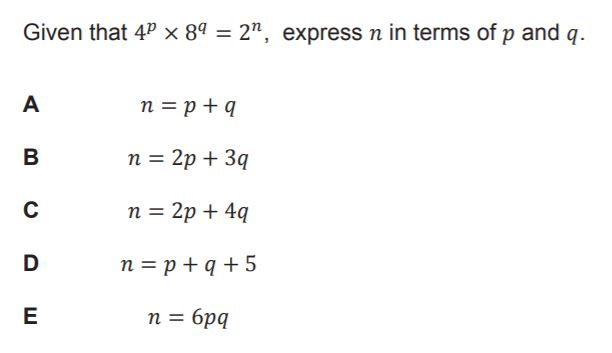### Algebra: Fully Worked Solution

This is a step-by-step explanation to this algebraic question.

Firstly, express 4 and 8 as powers of 2: 4 = 22, 8 = 23

Hence 4p x 8q = (22) p x (23) q = 22p x 23q = 22p+3q

Hence, n = 2p + 3q making the correct answer for this (B).

### Geometry: Fully Worked Question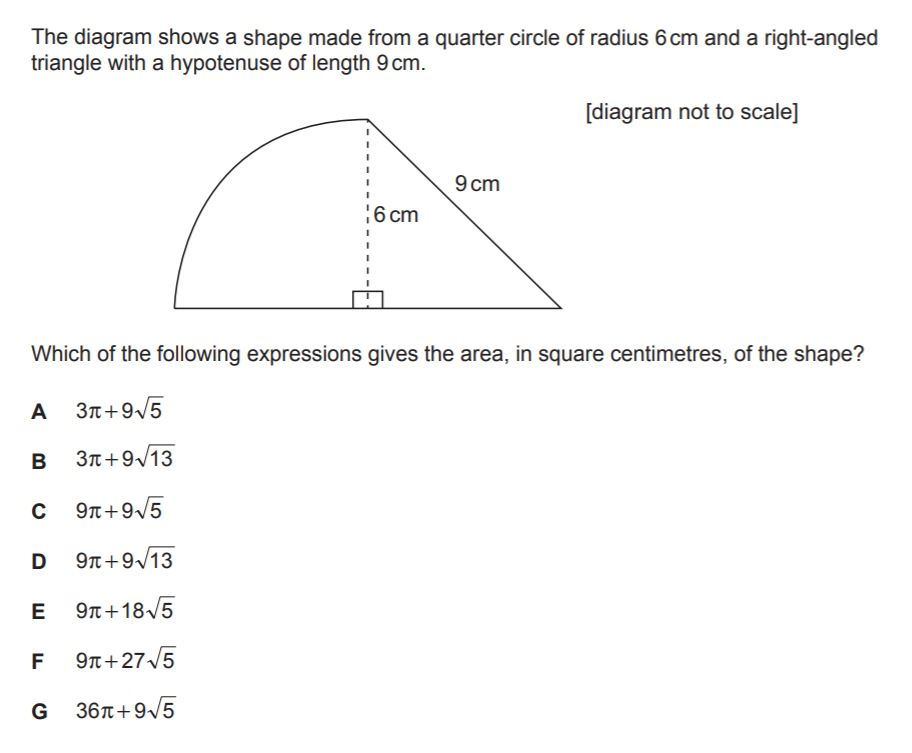### Geometry: Fully Worked Solution

This question tests you on knowing your geometrical formula and Pythagoras’s Theorem.

The area of the quarter circle formula is πr2 / 4 = 9π.

To find the area of the triangle, you must first find the missing side length using r2 + b2 = c2.

Therefore 92 = 62 + c2 which means c = √45.

In surd form, this can be expressed as 3√5.

Therefore the area of the triangle is 0.5 x 3√5 x 6 = 9√5.

### Statistics: Fully Worked Question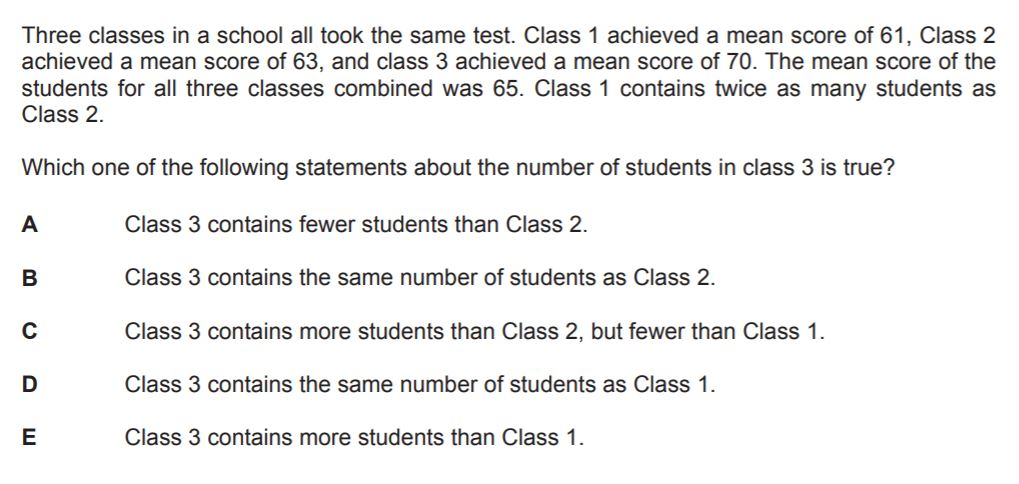### Statistics: Fully Worked Solution

As there are twice as many people in class 1 than 2, the total of all of the scores in Class 1 must be 61 × 2n = 122n.

The total of all of the scores in Class 2 must be 63n. If there are m students in Class 3, then the total of all the scores in Class 3 must be 70m.

The total score of all of the students must be 65 × (2n + n + m), so 195n + 65m = 122n + 63n + 70m.

This simplifies to 10n = 5m, so m = 2n.

The number of students in Class 3 is 2n, which is the same as the number of students in Class 1 (D).

This makes the correct answer to this question (D).

### Probability: Fully Worked Question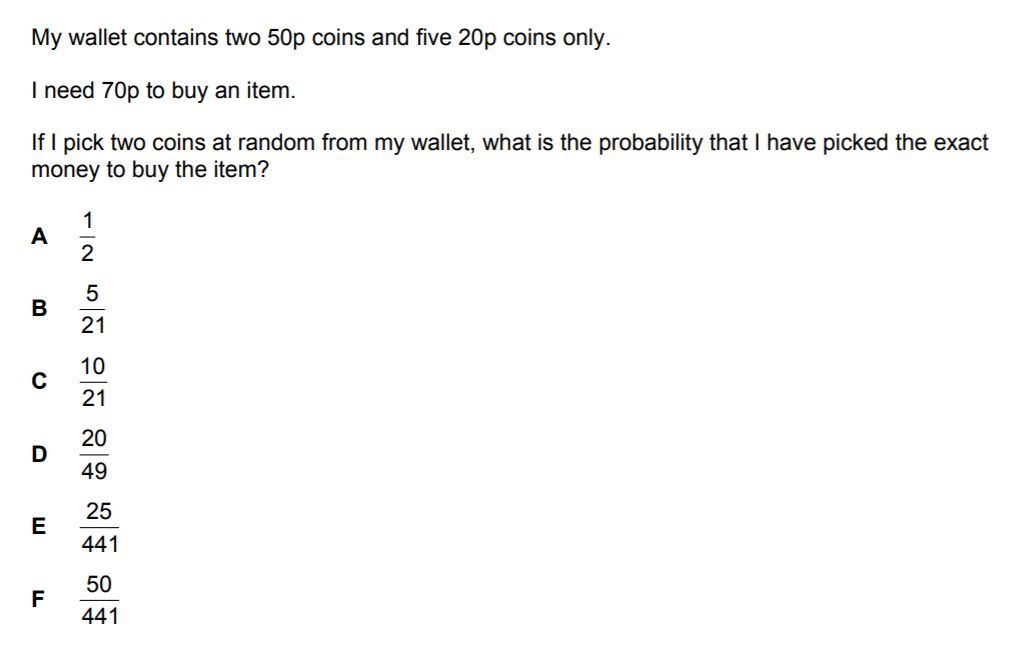### Probability: Fully Worked Solution

In this question, it might be an idea to draw a tree diagram.

The probability of picking a 20p then a 50p is {2/7 x 5/7) x 2.As well as the many different topics that you can be asked about, there are different formats of answers which you should be aware about.

1.Multiple Choice

The vast majority of Maths questions in section 2 are simply multiple choice as you can see in the examples used above. However, be wary of the questions and read it properly as there may be a few answers within the choices which might catch you out.

As a top tip, if you are unsure of an answer and running out of time, eliminate as many of the answers as you can using logic and then guess! Never leave a question unanswered.

Below is an example of a “multiple-choice” question (if you’re not sick of them just yet!).

The majority of questions you'll face are multiple-choice similar to the question above.

2.Which statements are correct

In section 2, there are questions which will provide you with statements and you must identify which statements are correct.

While these questions are rarely specifically about maths, they can be about Chemistry, Physics or Biology, which might require you to do some maths to find the answer.

Below is an example of a “which statement is correct” question.

This is a Physics question where maths is likely needed to come to the answer. You must choose which statements are correct.

If you are not completely sure… choose which statements you definitely know are correct, eliminate the other answers then guess from the remaining choices.

3. Tables

Lastly, some questions provide tables where you have to select which row is correct.

Like before, these questions are not typically asked within Maths but the format is still useful to know for the other sciences. Make sure you read each row properly and remember… eliminate and guess if you don’t know!

Below is an example of a “table” answer.

Again, this is a Physics questions however it illustrates the format of a table within an answer. Here, it is vital you check each row correctly before choosing an answer.

This is a Physics question where maths is likely needed to come to the answer. You must choose which statements are correct.

If you are not completely sure… choose which statements you definitely know are correct, eliminate the other answers then guess from the remaining choices.

## Closing Tips for the BMAT Maths section

1

Brush up on your mental maths.

As we have said before, timing is vital! You will need Maths in many questions in both Section 1 and Section 2. Therefore, make sure your mental maths is as good as it can be, as we promise it will save you a lot of time in the exam.

2

Practice, practice, practice!

There are many past papers available to you as well as question banks. The best way to find out what subjects you need to spend more time learning is to do these questions. We suggest doing as many papers as you can with a time limit, allowing you to get used to the time pressure.

3